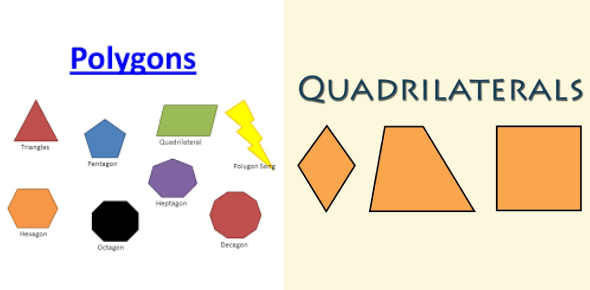# Polygons And Quadrilaterals Quiz! Test

19 Questions | Total Attempts: 941SettingsAre you familiar with polygons and quadrilaterals? Do you suppose you can take on this quiz? A quadrilateral is a polygon. It is a four-sided polygon, just like a triangle is a three-sided polygon. The five most commonplace quadrilaterals are the parallelogram, rectangle, square, trapezoid, and rhombus. Taking this quiz will put your knowledge about polygons and quadrilaterals to the test.

• 1.
A ___________________ has exactly one pair of parallel sides.
• 2.
A ______________ has four congruent sides and opposite sides are parallel.
• 3.
A ___________________ has four right angles, opposite sides are parallel, and opposite sides are congruent.
• 4.
A ________________  has four right angles and all sides are congruent.
• 5.
A square is a rhombus.
• A.

True

• B.

False

• 6.
A trapezoid is a parallelogram.
• A.

True

• B.

False

• 7.
A rectangle is a square.
• A.

True

• B.

False

• 8.
A square can be a rhombus and a rectangle.
• A.

True

• B.

False

• 9.
What is the polygon above?
• A.

Pentagon

• B.

Triangle

• C.

Hexagon

• 10.
What is the above polygon?
• A.

Decagon

• B.

Heptagon

• C.

Nonagon

• 11.
What is the above polygon?
• A.

Hexagon

• B.

• C.

Pentagon

• 12.
Tri means ___
• 13.
• 14.
Penta means ____
• 15.
Hexa means _____
• 16.
Hepta means ____
• 17.
Octa  means ____
• 18.
Nona means ______
• 19.
Deca means _____
Related TopicsBack to top